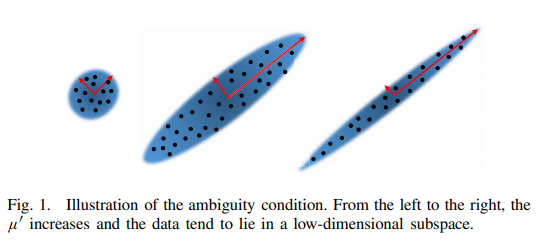# Completing Low-Rank Matrices with Corrupted Samples from Few Coefficients in General Basis### Abstract

Subspace recovery from the corrupted and missing data is crucial for various applications in signal processing and information theory. To complete missing values and detect column corruptions, the existing robust matrix completion (MC) methods mostly concentrate on recovering a low-rank matrix from a few corrupted coefficients with respect to standard basis, which, however, does not apply to more general basis, e.g., Fourier basis. In this paper, we prove that the range space of an m × n matrix with rank r can be exactly recovered from a few coefficients with respect to general basis, though r and the number of corrupted samples are both as high as O(min{m, n}/ log3(m + n)). Our model covers the previous ones as special cases, and robust MC can recover the intrinsic matrix with a higher rank. Moreover, we suggest a universal choice of the regularization parameter, which is λ = 1/√log n. By our ℓ2,1 filtering algorithm, which has theoretical guarantees, we can further reduce the computational cost of our model. As an application, we also find that the solutions to extended robust low-rank representation and to our extended robust MC are mutually expressible, so both our theory and algorithm can be applied to the subspace clustering problem with missing values under certain conditions. The experiments verify our theories.

Type
Publication
IEEE Trans. Information Theory